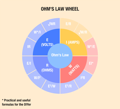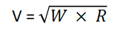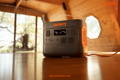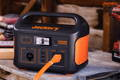## Ultimate Guide to Amps, Watts, and Volts With Jackery

Electricity has become a crucial part of our day-to-day life, and imagining living without power seems impossible. As solar energy is a safe, renewable, and clean alternative for homes, many people are investing in solar power stations.

However, it's worth noting the power station's amps, watts, voltage, etc., to ensure it can power appliances for long hours. Jackery Explorer Portable Power Stations are large-capacity solar solutions with high watts, volts, and amps values.In this guide, we will take a deep dive into electrical terms. We will focus on calculating voltage, watts, amps, etc., and on choosing the ideal-sized power station.

## What Are Amps, Watts, And Volts?

When understanding the nitty-gritty of electricity, it's crucial to learn basic electronic terms like amps, watts, voltage, and ohm.

Identifying the watt, ampere, voltage, and ohms for any power station is vital to confirming compatibility with your home or outdoor appliances. On the contrary, powering equipment using a wrong voltage battery is the primary reason for appliance operation failure.

The best part about these basic electrical terms is that they are interrelated and can be calculated using a simple formula. For example, you can calculate the watts an appliance consumes by multiplying amps and volts. Let us discuss how amps, watts, voltage, and ohms differ.

### Why Convert Watts to Amps?

Amperes, commonly called amps, measure the number of electrons flowing through a certain point per second. In other words, it describes the current flow through an electrical circuit.

In mathematical terms, amps are equal to watts divided by volts of a specific appliance.

Formula: Amps = Watts / Volts

If you have the watts and volts of an appliance, you can easily calculate its amps. For example, if the watts and volts of an electric device are 3600W and 240V, respectively, the amps value will be:

Amps = 3600W / 240V = 15A.

Amperage is the strength of the electric current and is expressed in amperes. The larger the amperage, the more electricity can flow through the circuit.

### What Is A Watt?

Wattage, commonly known as watts, is the power an electric appliance consumes. In other words, it is defined as the "electricity at work" or power a device takes to run.

In mathematical terms, watts are equal to the multiplication of amps and volts.

Formula: Watts = Amps × Volts

For instance, if an electric device uses 10 amps and 240 volts, the wattage will be:

Watts = 10 amps × 240 volts = 2400W.

The higher the wattage, the more the output and power of the appliance.

### What Is A Volt?

Volt measures the force or pressure required for an electric current to flow past a wire. In other words, it also determines the speed at which individual electrons flow through the circuit.

Large home appliances like washing machines, refrigerators, and air conditioners operate at 240V. On the contrary, smaller devices like computers, TVs, light bulbs, etc., operate at 120V.

In mathematical terms, volts are equal to the watts of an appliance divided by its amps.

Formula: Volts = Watts / Amps

If the appliance consumes 500 watts and 25 amps, the volts can be calculated as:

Volts = 500W / 25A = 20V

### What Is An Ohm?

The resistance inherent in an electric wire encountered by the electrons while flowing from high to low is called ohm.

In other words, it is the electrical resistance between two points of a conductor. When one volt of constant potential difference is applied to these points and produces a current of one ampere, the natural resistance equals one ohm.

Formula: Ohm = Volts / Amps

Or, Ω = V / A

For example, if there is 240V and 12A, the natural resistance of the conductor will be:

Ω = 240V / 12A = 20 Ω

## Amps, Watts, And Volts Relations

Electrical mathematics may seem overwhelming at first. But it's pretty simple once you dig deep. One of the simplest ways to understand the relation between amps, watts, and voltage is Ohm's Wheel Law.Let's take an example to help you understand how to use the wheel. Say you want to install an AC at your home and finalize a 4000-watt AC for your space. Now you want to determine the circuit required for AC to work efficiently.
In this case, we suppose the AC is rated at 4000 watts and must be supplied with 240 volts. As we already have watts and volts, we need to calculate amps or intensity of current (represented by I).
Now, choose the formula from Ohm's Wheel Law image.
I = W / E
Or, Amps = Watts ÷ Volts
Substitute the values:
Amps = 4000W ÷ 240V = 16.6 A.
As the AC will operate for 3 or 4 hours on a hot summer day, we apply a safety factor of 125%.
Amps = 16.6 × 1.25 = 20.8 amps.
That's it. A standard 25-amp circuit would supply stable and continuous electricity to the AC.
Voltage, Current, and Resistance
Let us understand how voltage (V), current (I), and resistance (R) relate to each other.
According to Ohm's law, they are related as follows:
V = I × R
Where,
V = Voltage (volts)
I = Current (amps)
R = Resistance (ohms)
That means increasing the voltage will directly increase the resistance when the current remains the same.
Note: Resistance works opposite to current. When the resistance is increased by keeping the same voltage, the current flowing through the circuit eventually decreases.
Power, Current, and Voltage
Now that you know how resistance, current, and voltage are related, here is a simple equation that connects power, current, and voltage.
P = V × I
Where,
P = Power (watts)
V = Voltage (volts)
I = Current (amps)
If you want to increase the power, consider increasing either current or voltage.

### Amps, Watts, And Volts Formulas

Now that you know the basics of wattage, amps, volts, and ohms, here are a few easy formulas to remember.
Amps Formulas:
The current (in amps) equals the voltage (in volts) divided by the resistance (in ohms).
I = V ÷ R
The current (in amps) equals the power (in watts) divided by the voltage (in volts).
I = W ÷ V
The current (in amps) equals the square root of power (in watts) divided by the resistance (in ohms).
I² = W ÷ R
Or, I =
Watts Formulas:
The power (in watts) equals the voltage (in volts) times the current (in amps).
W = V × I
The power (in watts) equals the square of voltage (in volts) divided by resistance (in ohms)
W = V² ÷ R
The power (in watts) equals the square of current (in amps) times the resistance (in ohms).
W = I² × R
Volts Formulas:
The voltage (in volts) equals the current (in amps) times the resistance (in ohms).
V = I × R
The voltage (in volts) equals the power (in watts) divided by the current (in amps).
V = W ÷ I
The voltage (in volts) equals the square root of power (in watts) times the resistance (in ohms).## Understand Amps, Watts, And Volts In the Electrical System

Besides watt, ampere, voltage, and ohms, there are a few additional electrical terms. Let us explain them briefly.
Atoms: They are known as the building blocks of matter that consist of a nucleus with protons and neutrons. It is surrounded by negatively charged electrons, resembling a mini solar system. Similar to atoms, the electrical system is made of matter.
Electrons: Unlike planets that move slowly, electrons move around the nucleus at the speed of light. The moving path of electrons is called a probability cloud.
Electricity: Atoms grouped together are called molecules. Both atoms and molecules located closely can easily pass electrons from one atom to another. This is the same case when electrons flow through a conductor.
Connecting your electrical equipment with the wrong voltage or power supply can cause appliance failure. That's why calculating the electrical ratings is essential before investing in a solar system or an electrical battery.

### The Electrical Efficiency

In an electrical system, power (measured in watts) equals the voltage multiplied by the current. Electrical efficiency means powering the same device using less energy. When you have an efficient electrical system, it means there is less waste of energy.
Suppose you are using a 12-volt battery and a 12V light bulb. You need 240 watts of power to light the bulb. Let us apply Ohm's Law to calculate the amps.
I = W ÷ V = 240W ÷ 12V = 20 amps.
Now let us say you have a 24-volt battery and a 24-volt light bulb to get the same amount of power, i.e., 240 watts.
I = W ÷ V = 240W ÷ 24V = 10 amps.
Resistance, in this case, will be 2.4 ohms.
Upon evaluating currents in the two scenarios, the latter system produces the same power using half the current. This means the second electrical system is more efficient.

## Jackery Power Stations Explained

While selecting the right power station, it's vital to note its amps, watts, and volts. The battery with higher watts can charge appliances for long hours, while high amperes mean it will charge your device faster.
Jackery is a renowned solar generator manufacturer offering high-quality portable power stations in different sizes. The portable, durable, and safe power stations are suitable for off-grid living, road trips, or camping.

### Jackery Explorer 2000 Pro Power Station

Featuring a 2160Wh battery and offering output power of 2200W, the Jackery Explorer 2000 Pro Portable Power Station can recharge up to eight electrical devices simultaneously. It can last up to 10 years, assuming you use the power station twice weekly.Capacity

Recharging Time

Ports

Appliances

2160Wh (43.2V/50Ah)

Explorer 2000 Pro + 6*SolarSaga 100W: 5.5H

2*AC Output: 230V, 2200W (peak 4400W); 2*USB-A: Quick Charge 3.0x2, 18W Max; 2*USB-C: 100W Max, (5V, 9V, 15V, 12V, 20V up to 5A); 1*Carport: 12V⎓10A

TV(200W): 8.4H

Refrigerator(700W): 2.4H

Microwave(750W): 2.2H

Coffee Maker(800W): 2.1H

Blender(300W): 5.6H

Stove(850W): 2H

### Jackery Explorer 1000 Pro Power Station

The fully-compatible lithium-ion battery has a capacity of 1002Wh that can charge 93% of home or outdoor appliances. It weighs only 25.4 lbs, making this power station a portable charging solution for outdoor activities.Capacity

Recharging Time

Ports

Appliances

1002Wh (43.2V, 23.2Ah)

Explorer 1000 Pro + 6*SolarSaga 80W: 9H

2*AC Output: 230V, 1000W, peak 2000W; 2*USB-A: Quick Charge 3.0x2, 18W Max; 2*USB-C: 100W Max, (5V, 9V, 15V, 12V, 20V up to 5A); 1*Carport: 12V⎓10A

TV(200W): 4H

Fridge(500W): 1.6H

Blender(300W): 2.7H

CPAP Machine(100W): 8H

Computer(200W): 4H

Electric Wheelchair(380W): 2.1H

### Jackery Explorer 500 Power Station

It is portable and lightweight, measuring precisely the same dimensions as a basketball, making it simple to transport. The SolarSaga 100W solar panel is foldable, mobile, and light at 4.69 kilograms; it even has an easy-carry handle. A quiet home generator will not disrupt your slumber, unlike conventional gas generators. The 500 dB output of the Solar Generator makes it an exceptionally silent generator for camping.Capacity

Recharging Time

Ports

Appliances

518Wh (21.6V, 24Ah)

Explorer 500 + 1*SolarSaga 100W: 9.5H

1*AC Output: 240V, 500W (peak 1000W); 2*DC Output: 12V⎓7A; 3*USB-A: 5V⎓2.4A; 1*Carport: 12V⎓10A

Phone(10W): 44H

TV(60W): 7.3H

Fan(100W): 4.4H

Lighting(25W): 17.6H

Speaker(120W): 3.7H

Computer(200W): 2.2H

## Amps, Watts, And Volts FAQs

1. How many amps are in 10 watts at 120 volts?

You can calculate the amps by dividing the given watts by volts.

Amps = Watts ÷ Volts = 10W ÷ 120V = 0.083A

Here we have a few more ampere calculations with different power and volts.

Power (W)

Voltage (V)

Current (A)

10 Watts

120 Volts

0.0833 amps

20 Watts

120 Volts

0.167 amps

30 Watts

120 Volts

0.250 amps

40 Watts

120 Volts

0.333 amps

50 Watts

120 Volts

0.417 amps

60 Watts

120 Volts

0.500 amps

70 Watts

120 Volts

0.583 amps

2. Does higher watts mean more power?

In short, yes. As watts represent the rate at which energy is used or transferred, it is directly related to power. The higher the watts of a battery capacity, the more power.

3. What are the differences between amps, watts, and volts? Is watts stronger than volts?

Here is a brief definition of wattage, amps, volts, etc.

Amps measure the electrical current or the speed at which electrons flow through a conductor.

Volts measure the electrical voltage and represent the difference in electrical potential.

Watts is the rate at which electrical energy is transferred in a circuit.

Watts measure power, while volts represent electrical potential. That being said, the energy used or transferred is calculated in watts. Therefore, watts are stronger than volts.

## Final Thoughts

Knowing the current flowing the load is vital in choosing the best wire. The amps, watts, voltage, and ohms formulas are also helpful in calculating the size of the solar inverter and total power consumption. Once you know how much power your appliances consume, you can easily calculate the portable power station size.

Depending on your power needs, you can choose Jackery Explorer Portable Power Stations, available in different sizes. If you want to power all small and large home or outdoor appliances for hours, consider investing in the ultimate power solution Jackery Explorer 2000 Pro Portable Power Station.

### New Arrival

Hurry up! Sale ends once the timer hits zero

00Days
00Hrs
00Mins
00Secs

### Best-selling Jackery Portable Power Stations

Sale Off### Jackery Explorer 2000 Plus Portable Power Station

\$3,599.00\$3,419.00
Sale Off### Jackery Explorer 2000 Pro Portable Power Station

\$3,299.00
Sale Off### Jackery Explorer 1000 Pro Portable Power Station

\$1,999.00\$1,699.00